Ch.4 + 5 - Statistics, Quality Assurance and Calibration MethodsWorksheetSee all chapters
 Ch.1 - Chemical Measurements 2hrs & 8mins 0% complete Worksheet Ch.2 - Tools of the Trade 1hr & 26mins 0% complete Worksheet Ch.3 - Experimental Error 1hr & 53mins 0% complete Worksheet Ch.4 + 5 - Statistics, Quality Assurance and Calibration Methods 2hrs & 1min 0% complete Worksheet Ch.6 - Chemical Equilibrium 3hrs & 43mins 0% complete Worksheet Ch.7 - Activity and the Systematic Treatment of Equilibrium 1hr & 1min 0% complete Worksheet Ch.8 - Monoprotic Acid-Base Equilibria 1hr & 52mins 0% complete Worksheet Ch.9 - Polyprotic Acid-Base Equilibria 2hrs & 16mins 0% complete Worksheet Ch.10 - Acid-Base Titrations 2hrs & 40mins 0% complete Worksheet Ch.11 - EDTA Titrations 1hr & 37mins 0% complete Worksheet Ch.12 - Advanced Topics in Equilibrium 1hr & 18mins 0% complete Worksheet Ch.13 - Fundamentals of Electrochemistry 2hrs & 27mins 0% complete Worksheet Ch.14 - Electrodes and Potentiometry 43mins 0% complete Worksheet Ch.15 - Redox Titrations 1hr & 14mins 0% complete Worksheet Ch.16 - Electroanalytical Techniques 1hr & 3mins 0% complete Worksheet Ch.17 - Fundamentals of Spectrophotometry 55mins 0% complete Worksheet BONUS: Chemical Kinetics Not available yet

# The Gaussian Distribution

See all sections
Sections
Mean Evaluation
The Gaussian Distribution
Confidence Intervals
Hypothesis Testing (t-Test)
Analysis of Variance (f-Test)
Detection of Gross Errors

Performing an experiment numerous times with no systematic error results in a smooth curve called the Gaussian Distribution.

###### The Gaussian Distribution & Z-Table

Concept #1: Understanding the Gaussian Distribution Curve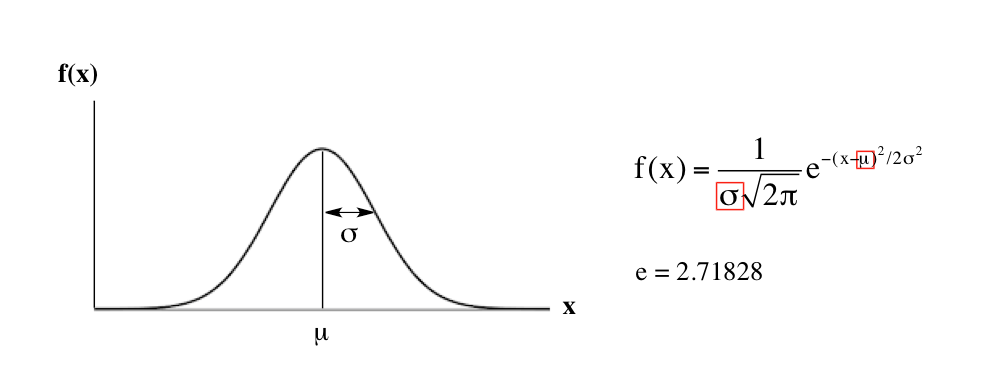Example #1: Understanding standard normal distribution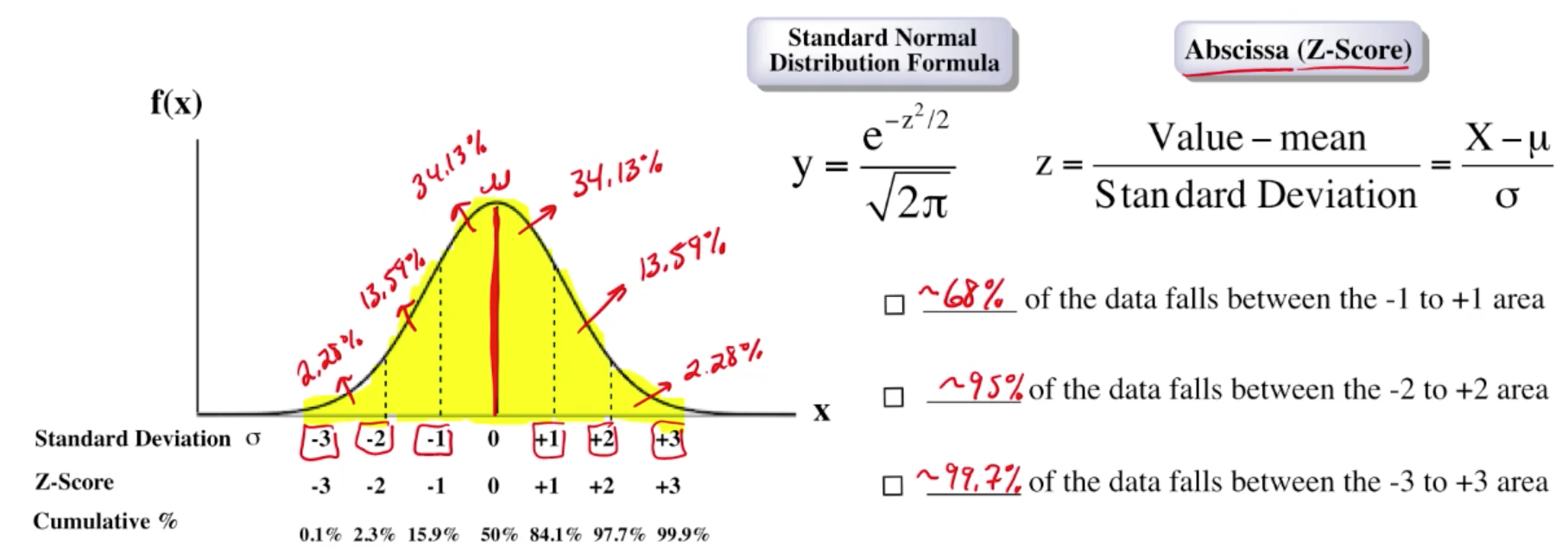Example #2: Understanding Z-Tables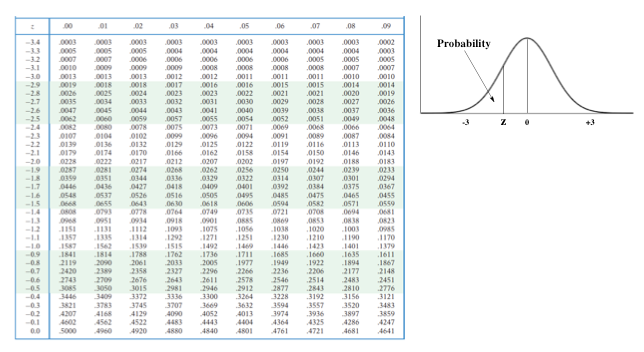Example #3: The use of Z-Tables is essential in the determination of probabilities.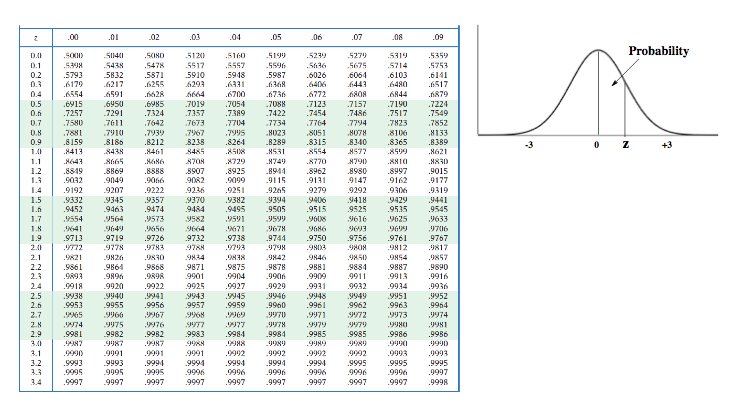###### The Gaussian Distribution & Z-Tables Calculations

Example #4: Suppose there are 100 students in your analytical lecture and at the end of the semester the class average is an 80 with a standard deviation of 5.3, determine the distribution and probability of grades based on your understanding of the Gaussian distribution curve.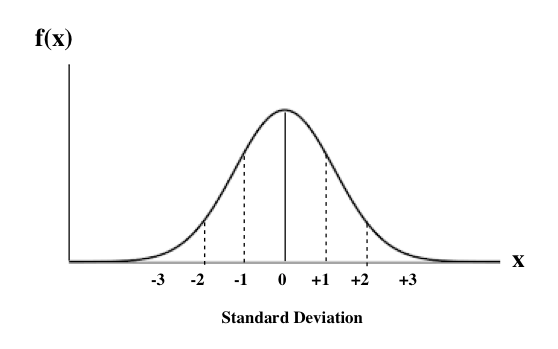Example #5: From EXAMPLE 1, determine the percentage of final grades that would lie below 71.

Practice: From EXAMPLE 1, determine the percentage of final grades that would lie between 88 to 92.